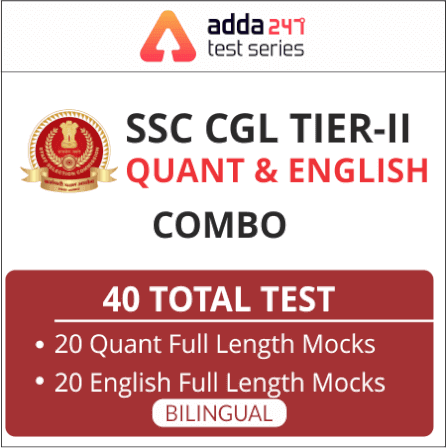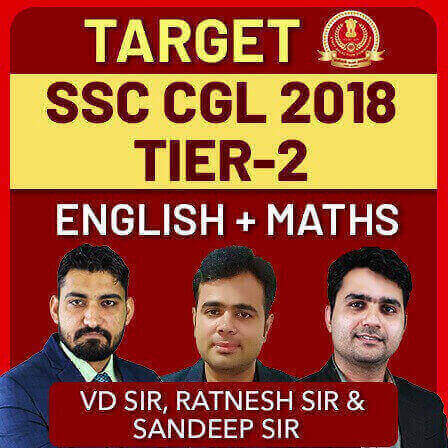# SSC CGL Mains Co-ordinate Geometry Questions : 3rd July

Dear aspirants,

As you all know, the upcoming months are lined up with various important exams like SSC CGL Mains 2018, so we are here to help you with the subject that is common to all of the given exams. We are providing daily quantitative aptitude quizzes, practice which will help you to score good marks in this section. We aim to provide the best study material to our readers with exam level questions to help them get used to the recent pattern. Attempt this quiz and check your preparation.

SSC CGL 2018-19 Expected Cutoff | Comparison with Previous YearsQ5. If (–5, 4) divides the line segment between the coordinate axes in the ratio 1 : 2, then what is its equation?
यदि (-5, 4) समायोजन ध्रुव के बीच रेखा खंड को 1: 2 के अनुपात में विभाजित करता है, तो इसका समीकरण क्या है?
(a) 8x + 5y + 20 = 0
(b) 5x + 8y – 7 = 0
(c) 8x – 5y + 60 = 0
(d) 5x – 8y + 57 = 0Q6. What is the locus of a point which is equidistant from the point (m + n, n – m) and the point (m – n, n + m) ?
एक बिंदु का स्थान क्या है जो बिंदु (m + n, n – m) और बिंदु (m – n, n + m) से समान दूरी पर है?
(a) mx = ny
(b) nx = – my
(c) nx = my
(d) mx = – nyQ7. If the three vertices of the parallelogram ABCD are A(1, a), B(3, a), C(2, b), then D is equal to?
यदि समांतर चतुर्भुज ABCD के तीन कोने A (1,a), B (3, a), C (2, b) हैं, तो D किस के बराबर है?
(a) (3, b)
(b) (0, b)
(c) (4, b)
(d) (5, b)

S7. Ans.(b)

Q8. The area of a triangle, whose vertices are (3, 4), (5, 2) and the point of intersection of the lines x = a and y = 5, is 3 square units. What is the value of a?
एक त्रिभुज का क्षेत्रफल, जिसके कोने (3, 4), (5, 2) और रेखाओं के प्रतिच्छेदन बिंदु x = a और y = 5 हैं, 3 वर्ग इकाई है। a का मान क्या है?
(a) 2
(b) 3
(c) 4
(d) 5#### SSC CGL Syllabus 2019: Check SSC CGL Syllabus for All Tiers

You May Also Like To Read :